GMAT Math : Calculating whether quadrilaterals are similar

Example Questions

2 Next →

Example Question #461 : Problem Solving Questions

In Quadrilateral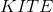,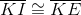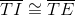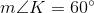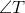is a right angle.

There exists Quadrilateral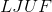such that Quadrilateral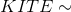Quadrilateral, and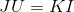.

Which of the following is true about the areas of the two quadrilaterals?

Quadrilateraland Quadrilateralhave the same area.

Quadrilateralhas area three times that of Quadrilateral.

Quadrilateralhas area three times that of Quadrilateral.

Quadrilateralhas area twice that of Quadrilateral.

Quadrilateralhas area twice that of Quadrilateral.

Quadrilateralhas area twice that of Quadrilateral.

Explanation:

We will assume that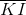and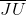have common measure 1 for the sake of simplcity; this reasoning is independent of the actual measure of.

The Quadrilateralwith its diagonals is shown below. We call the point of intersection: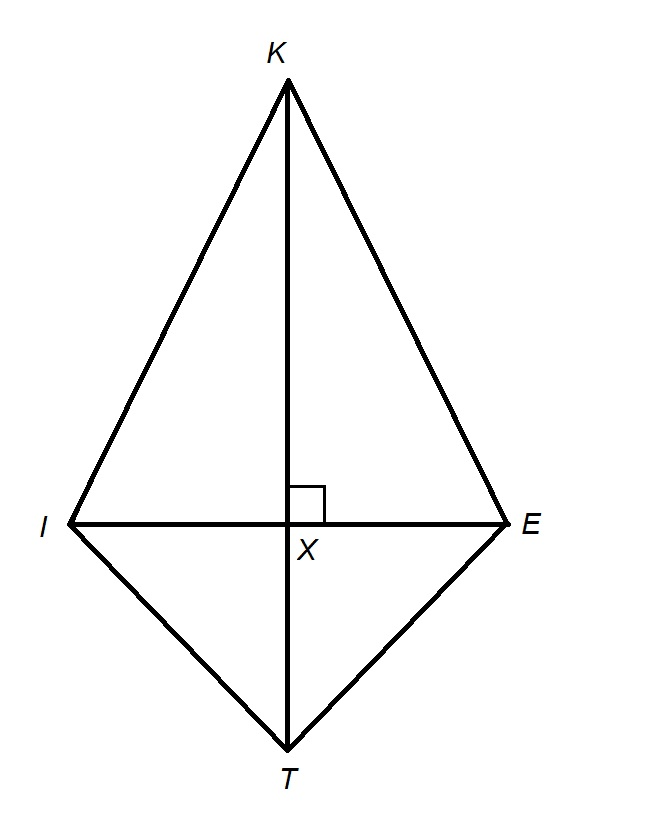The diagonals of a quadrilateral with two pairs of adjacent congruent sides - a kite - are perpendicular; also,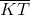bisects theandangles of the kite. Consequently,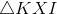is a 30-60-90 triangle and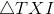is a 45-45-90 triangle. By the 30-60-90 Theorem, since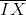andare the short leg and hypotenuse of,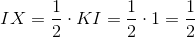.

By the 45-45-90 Theorem, sinceand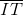are a leg and the hypotenuse of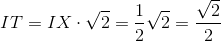The similarity ratio of Quadrilateralto Quadrilateralcan be found by finding the ratio of the length of sideto corresponding side: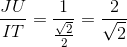The ratio of the areas is the square of the similarity ratio: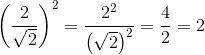The correct choice is that Quadrilateralhas area twice that of Quadrilateral.

2 Next →

Tired of practice problems?

Try live online GMAT prep today.